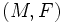Flag curvature

The flag curvature of a Finsler manifold$(M,F)$ (viz a differential manifold$M$ endowed with a Finsler metric$F$) defines a scalar (real number) for the following input: a point on the manifold, a tangent plane at that point, and a nonzero vector in the tangent plane.